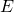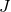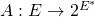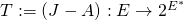# Explicit algorithms for J-fixed points of some non linear mappings in certain Banach spaces

### Full PDFcreative_2020_29_1_27_36

Letbe a real normed linear space andits dual. In a recent work, Chidume et al. [ Chidume, C. E. and Idu, K. O., Approximation of zeros of bounded maximal monotone mappings, solutions of hammerstein integral equations and convex minimizations problems, Fixed Point Theory and Applications,  97
(2016)] introduced the new concepts of-fixed points andpseudocontractive mappings and they shown that a mappingis monotone if and only if the mapispseudocontractive, whereis the normalized duality mapping of. It is our purpose in this work to introduce an algorithm for approximating-fixed points ofpseudocontractive mappings. Our results are applied to approximate zeros of monotone mappings in certain Banach spaces. The results obtained here, extend and unify some recent results in this direction for the class of maximal monotone mappings in uniformly smooth and strictly convex real Banach spaces. Our proof is of independent interest.